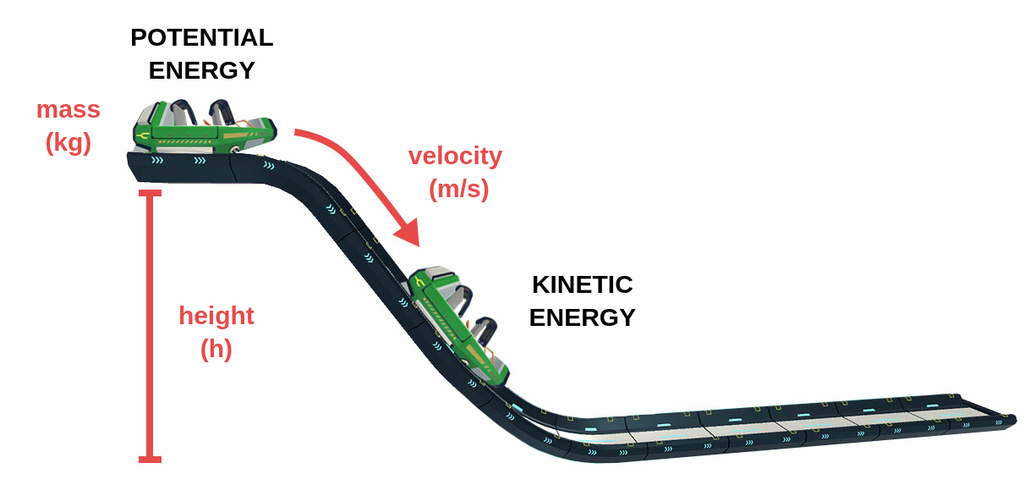Potential energy is an object's energy due to its position relative to other objects or stresses within itself, among other factors.

One common example of potential energy is gravitational potential energy near Earth's surface. It is the energy an object has due to its relative position above the Earth's center. Gravitational potential energy, as shown by the formula below, is the product of mass (m), gravitational acceleration (g, on Earth = 9.8 m/s2), and height (h). Potential energy is measured in joules (kg·m2/s2).

### EP = m g h

Together, potential energy and kinetic energy make up mechanical energy.Figure 1: Potential and Kinetic Energy